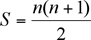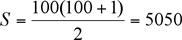Name: jo Who is asking: Student Level of the question: Secondary Question: what is the sum of the first 100 whole numbers?? how am i supposed to work this out efficiently? thanks Hi Jo, The question you asked relates back to a famous mathematician, Gauss.  In elementary school in the late 1700’s, Gauss was asked to find the sum of the numbers from 1 to 100.  The question was assigned as “busy work” by the teacher, but Gauss found the answer rather quickly by discovering a pattern.  His observation was as follows: 1 + 2 + 3 + 4 + … + 98 + 99 + 100 Gauss noticed that if he was to split the numbers into two groups (1 to 50 and 51 to 100), he could add them together vertically to get a sum of 101. 1     + 2   + 3   + 4   + 5   + … + 48 + 49 + 50 100 + 99 + 98 + 97 + 96 + … + 53 + 52 + 51 1 + 100 = 101 2 + 99 = 101 3 + 98 = 101 . . . 48 + 53 = 101 49 + 52 = 101 50 + 51 = 101 Gauss realized then that his final total would be 50(101) = 5050. The sequence of numbers (1, 2, 3, … , 100) is arithmetic and when we are looking for the sum of a sequence, we call it a series.  Thanks to Gauss, there is a special formula we can use to find the sum of a series:S is the sum of the series and n is the number of terms in the series, in this case, 100.Hope this helps! There are other ways to solve this problem. You can, for example, memorize the formula This is an arithmetic series, for which the formula is: S = n[2a+(n-1)d]/2 where a is the first term, d is the difference between terms, and n is the number of terms. For the sum of the first 100 whole numbers: a = 1, d = 1, and n = 100 Therefore, sub into the formula: S = 100[2(1)+(100-1)(1)]/2 = 100/2 = 5050 You can also use special properties of the particular sequence you have. An advantage of using Gauss' technique is that you don't have to memorize a formula, but what do you do if there are an odd number of terms to add so you can't split them into two groups, for example "what is the sum of the first 21 whole numbers?" Again we write the sequence "forwards and backwards" but using the entire sequence. 1   +  2  +  3 + ... + 19 + 20 + 21 21 + 20 + 19 + ... +   3 +   2 +  1 Now if you add vertically you get 22 + 22 + 22 + ... + 22 + 22 + 22 = 21(22) = 462 But this is twice the sum of the first 21 whole numbers so 1 + 2 + 3 + ... + 19 + 20 + 21 = 462/2 = 231 Natasha, Paul and Penny